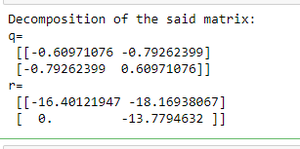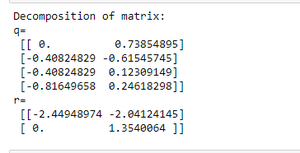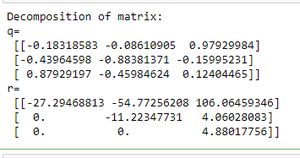# Get the QR factorization of a given NumPy array

• Last Updated : 29 Aug, 2020

In this article, we will discuss QR decomposition or QR factorization of a matrix. QR factorization of a matrix is the decomposition of a matrix say ‘A’ into ‘A=QR’ where Q is orthogonal and R is an upper-triangular matrix. We factorize the matrix using numpy.linalg.qr() function.

Syntax : numpy.linalg.qr(a, mode=’reduced’)

Attention geek! Strengthen your foundations with the Python Programming Foundation Course and learn the basics.

To begin with, your interview preparations Enhance your Data Structures concepts with the Python DS Course. And to begin with your Machine Learning Journey, join the Machine Learning - Basic Level Course

Parameters :

• a : matrix(M,N) which needs to be factored.
• mode : it is optional. It can be :

Below are some examples of how to use the above-described function :

Example 1: QR factorization of 2X2 matrix

## Python3

 `# Import numpy package``import` `numpy as np`` ` `# Create a numpy array ``arr ``=` `np.array([[``10``,``22``],[``13``,``6``]])`` ` `# Find the QR factor of array``q, r ``=`  `np.linalg.qr(arr)`` ` `# Print the result``print``(``"Decomposition of matrix:"``)``print``( ``"q=\n"``, q, ``"\nr=\n"``, r)`

Output :Example 2: QR factorization of 2X4 matrix

## Python3

 `# Import numpy package``import` `numpy as np`` ` `# Create a numpy array ``arr ``=` `np.array([[``0``, ``1``], [``1``, ``0``], [``1``, ``1``], [``2``, ``2``]])`` ` `# Find the QR factor of array``q, r ``=`  `np.linalg.qr(arr)`` ` `# Print the result``print``(``"Decomposition of matrix:"``)``print``( ``"q=\n"``, q, ``"\nr=\n"``, r)`

Output :Example 3: QR factorization of 3X3 matrix

## Python3

 `# Import numpy package``import` `numpy as np`` ` `# Create a numpy array ``arr ``=` `np.array([[``5``, ``11``, ``-``15``], [``12``, ``34``, ``-``51``],``                ``[``-``24``, ``-``43``, ``92``]], dtype``=``np.int32)`` ` `# Find the QR factor of array``q, r ``=` `np.linalg.qr(arr)`` ` `# Print the result``print``(``"Decomposition of matrix:"``)``print``( ``"q=\n"``, q, ``"\nr=\n"``, r)`

Output :My Personal Notes arrow_drop_up